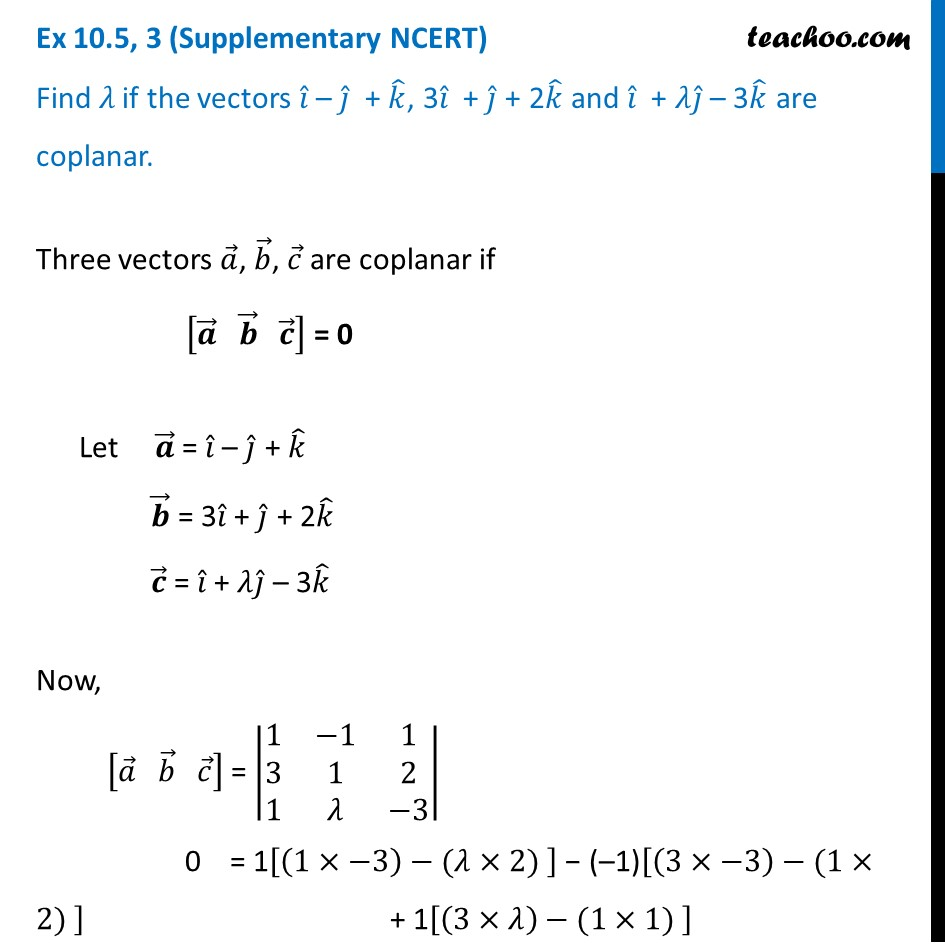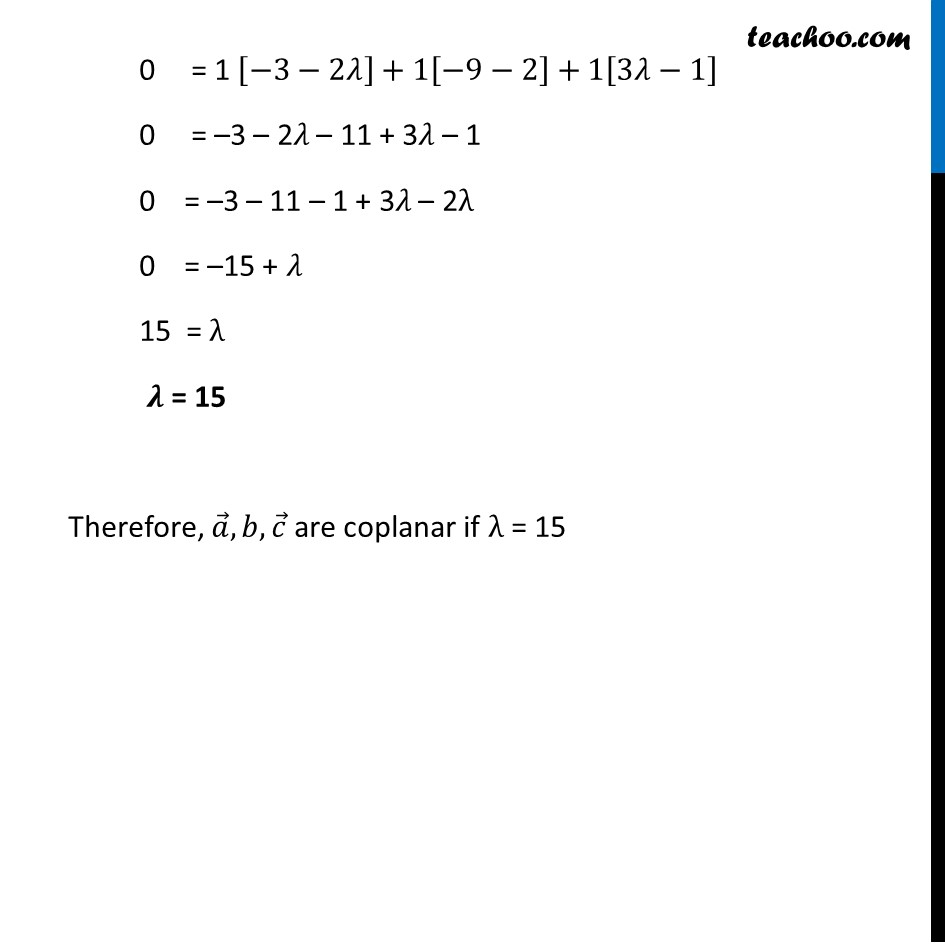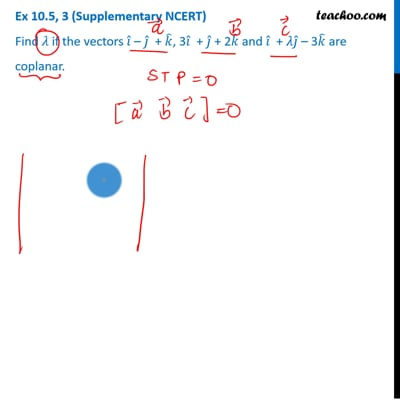Ex 10.5 (Supplementary NCERT)

Chapter 10 Class 12 Vector Algebra
Serial order wiseThis video is only available for Teachoo black users

Introducing your new favourite teacher - Teachoo Black, at only ₹83 per month

### Transcript

Ex 10.5, 3 (Supplementary NCERT) Find 𝜆 if the vectors 𝑖 ̂ – 𝑗 ̂ + 𝑘 ̂, 3𝑖 ̂ + 𝑗 ̂ + 2𝑘 ̂ and 𝑖 ̂ + 𝜆𝑗 ̂ – 3𝑘 ̂ are coplanar.Three vectors 𝑎 ⃗, 𝑏 ⃗, 𝑐 ⃗ are coplanar if [𝒂 ⃗" " 𝒃 ⃗" " 𝒄 ⃗ ] = 0 Let 𝒂 ⃗ = 𝑖 ̂ – 𝑗 ̂ + 𝑘 ̂ 𝒃 ⃗ = 3𝑖 ̂ + 𝑗 ̂ + 2𝑘 ̂ 𝒄 ⃗ = 𝑖 ̂ + 𝜆𝑗 ̂ – 3𝑘 ̂ Now, [𝑎 ⃗" " 𝑏 ⃗" " 𝑐 ⃗ ] = |■8(1&−1&1@3&1&2@1&"𝜆" &−3)| 0 = 1[(1×−3)−("𝜆" ×2) ] − (–1)[(3×−3)−(1×2) ] + 1[(3×"𝜆" )−(1×1) ] 0 = 1 [−3−2"𝜆" ]+1[−9−2]+1[3"𝜆" −1] 0 = –3 – 2𝜆 – 11 + 3𝜆 – 1 0 = –3 – 11 – 1 + 3𝜆 – 2λ 0 = –15 + 𝜆 15 = λ 𝝀 = 15 Therefore, 𝑎 ⃗,𝑏,𝑐 ⃗ are coplanar if λ = 15